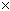SEARCH HOMEMath Central Quandaries & QueriesQuestion from Debbie: We are wanting to pour a 24'x30' slab that is 5" deep. How many cubic yards of cement do we need? Thanks!Hi Debbie,

24 ft is 24/3 = 8 yards
30 ft is 30/3 = 10 yards
5 inches is 5/36 yards.

Thus the volume is

8105/36 = 11.1 cubic yards.

You can calculate this and similar volumes using our volume calculator.

Penny

Volume Calculator

Use this calculator to find the amount of concrete, cement, topsoil or water you need for a rectangular solid shape when you know the length, width and depth.

 Length: meters centimeters yards feet inches Width: meters centimeters yards feet inches Depth: meters centimeters yards feet inches

Most often, when you order concrete or large amounts of topsoil, orders and deliveries are done in cubic yards (which are often just called "yards").Math Central is supported by the University of Regina and The Pacific Institute for the Mathematical Sciences.